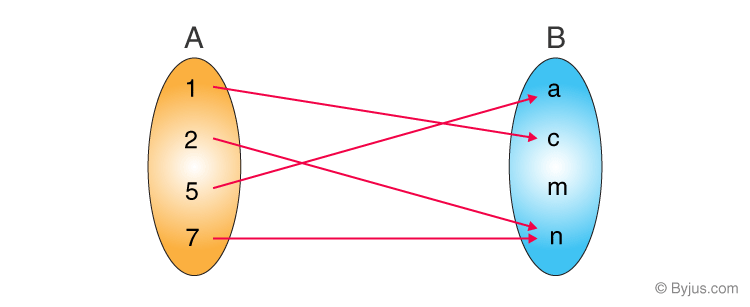# Relations And Its Types

Relations and its types concepts are one of the important topics of set theory. Sets, relations and functions all three are interlinked topics. Sets denote the collection of ordered elements whereas relations and functions define the operations performed on sets.

The relations define the connection between the two given sets. Also, there are types of relations stating the connections between the sets. Hence, here we will learn about relations and their types in detail.

## Relations Definition

A relation in mathematics defines the relationship between two different sets of information. If two sets are considered, the relation between them will be established if there is a connection between the elements of two or more non-empty sets.

In the morning assembly at schools, students are supposed to stand in a queue in ascending order of the heights of all the students. This defines an ordered relation between the students and their heights.

Therefore, we can say,

‘A set of ordered pairs is defined as a relation.’This mapping depicts a relation from set A into set B. A relation from A to B is a subset of A x B. The ordered pairs are (1,c),(2,n),(5,a),(7,n). For defining a relation, we use the notation where,

set {1, 2, 5, 7} represents the domain.

set {a, c, n} represents the range.

## Sets and Relations

Sets and relation are interconnected with each other. The relation defines the relation between two given sets.

If there are two sets available, then to check if there is any connection between the two sets, we use relations.

For example, an empty relation denotes none of the elements in the two sets is same.

Let us discuss the other types of relations here.

## Relations in Mathematics

In Maths, the relation is the relationship between two or more set of values.
Suppose, x and y are two sets of ordered pairs. And set x has relation with set y, then the values of set x are called domain whereas the values of set y are called range.
Example: For ordered pairs={(1,2),(-3,4),(5,6),(-7,8),(9,2)}
The domain is = {-7,-3,1,5,9}
And range is = {2,4,6,8}

## Types of Relations

There are 8 main types of relations which include:

• Empty Relation
• Universal Relation
• Identity Relation
• Inverse Relation
• Reflexive Relation
• Symmetric Relation
• Transitive Relation
• Equivalence Relation

### Empty Relation

An empty relation (or void relation) is one in which there is no relation between any elements of a set. For example, if set A = {1, 2, 3} then, one of the void relations can be R = {x, y} where, |x – y| = 8. For empty relation,

R = φ ⊂ A × A

### Universal Relation

A universal (or full relation) is a type of relation in which every element of a set is related to each other. Consider set A = {a, b, c}. Now one of the universal relations will be R = {x, y} where, |x – y| ≥ 0. For universal relation,

R = A × A

### Identity Relation

In an identity relation, every element of a set is related to itself only. For example, in a set A = {a, b, c}, the identity relation will be I = {a, a}, {b, b}, {c, c}. For identity relation,

I = {(a, a), a ∈ A}

### Inverse Relation

Inverse relation is seen when a set has elements which are inverse pairs of another set. For example if set A = {(a, b), (c, d)}, then inverse relation will be R-1 = {(b, a), (d, c)}. So, for an inverse relation,

R-1 = {(b, a): (a, b) ∈ R}

### Reflexive Relation

In a reflexive relation, every element maps to itself. For example, consider a set A = {1, 2,}. Now an example of reflexive relation will be R = {(1, 1), (2, 2), (1, 2), (2, 1)}. The reflexive relation is given by-

(a, a) ∈ R

### Symmetric Relation

In a symmetric relation, if a=b is true then b=a is also true. In other words, a relation R is symmetric only if (b, a) ∈ R is true when (a,b) ∈ R. An example of symmetric relation will be R = {(1, 2), (2, 1)} for a set A = {1, 2}. So, for a symmetric relation,

aRb ⇒ bRa, ∀ a, b ∈ A

### Transitive Relation

For transitive relation, if (x, y) ∈ R, (y, z) ∈ R, then (x, z) ∈ R. For a transitive relation,

aRb and bRc ⇒ aRc ∀ a, b, c ∈ A

### Equivalence Relation

If a relation is reflexive, symmetric and transitive at the same time, it is known as an equivalence relation.

## Video Lesson

### Types of Relations## Representation of Types of Relations

Relation Type Condition
Empty Relation R = φ ⊂ A × A
Universal Relation R = A × A
Identity Relation I = {(a, a), a ∈ A}
Inverse Relation R-1 = {(b, a): (a, b) ∈ R}
Reflexive Relation (a, a) ∈ R
Symmetric Relation aRb ⇒ bRa, ∀ a, b ∈ A
Transitive Relation aRb and bRc ⇒ aRc ∀ a, b, c ∈ A

## Recommended Videos

### Relations and Types of Relations### Functions – Introduction, Co-domain and RangeTest your knowledge on Relations And Its Types